• java符合运算符
2021-03-13 15:32:12

1.1 逻辑运算符

逻辑运算符，它是用于布尔值进行运算的，运算的最终结果为布尔值true或false。

运算符

运算规则

范例

结果

&

false&true

False

|

false|true

True

^

异或

true^flase

True

!

!true

Flase

&&

短路与

false&&true

False

||

短路或

false||true

True

看完图后，我们来看一下逻辑运算符的常规使用方式：

l 逻辑运算符通常连接两个其他表达式计算后的布尔值结果

l 当使用短路与或者短路或时，只要能判断出结果则后边的部分就不再判断。

int x = 1,y = 1;

if(x++==2 & ++y==2)

{

x =7;

}

System.out.println("x="+x+",y="+y);

&与，把条件都走一遍，不管结果的对与错。当条件都满足时才会进入循环体。

int x = 1,y = 1;

if(x++==2 && ++y==2)

{

x =7;

}

System.out.println("x="+x+",y="+y);

&&短路与，一短路就不走了，如果第一个结果是false，那后面的就不走了，直接结束(跳出循环)，如果不是接着往下走。

int x = 1,y = 1;

if(x++==1 | ++y==1)

{

x =7;

}

System.out.println("x="+x+",y="+y);

|或，只要有一个条件满足就进循环体，并且走完整个条件

int x = 1,y = 1;

if(x++==1 || ++y==1)

{

x =7;

}

System.out.println("x="+x+",y="+y);

||短路或，只要有一个符合条件就不走后面的，如第一个符合条件，不走后面，直接进入循环体。

更多相关内容
• 自增自减运算符(练习) //自增自减运算符 y的值是多少? int x = 10; int y = x++ ＋ x++ + x++; System.out.println(y); 自增自减运算符(练习) //自减运算符 //b的值是多少? int a = 10; int b=a--; System.out....

# 上期知识回顾

自增自减运算符(练习)

//自增自减运算符
y的值是多少?
int x = 10;
int y = x++ ＋ x++ + x++;
System.out.println(y);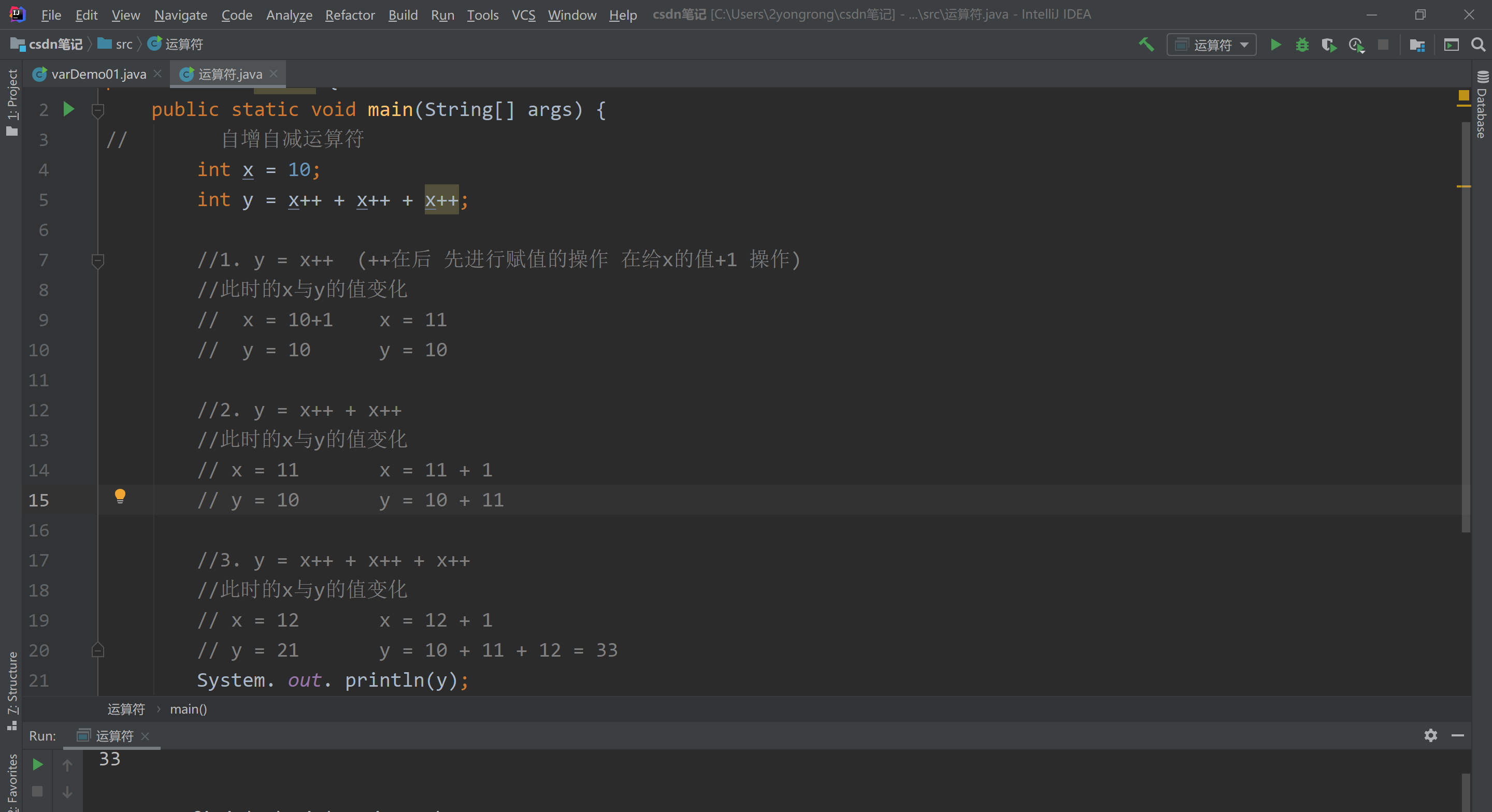自增自减运算符(练习)

//自减运算符
//b的值是多少?
int a = 10;
int b=a--;
System.out.println(b);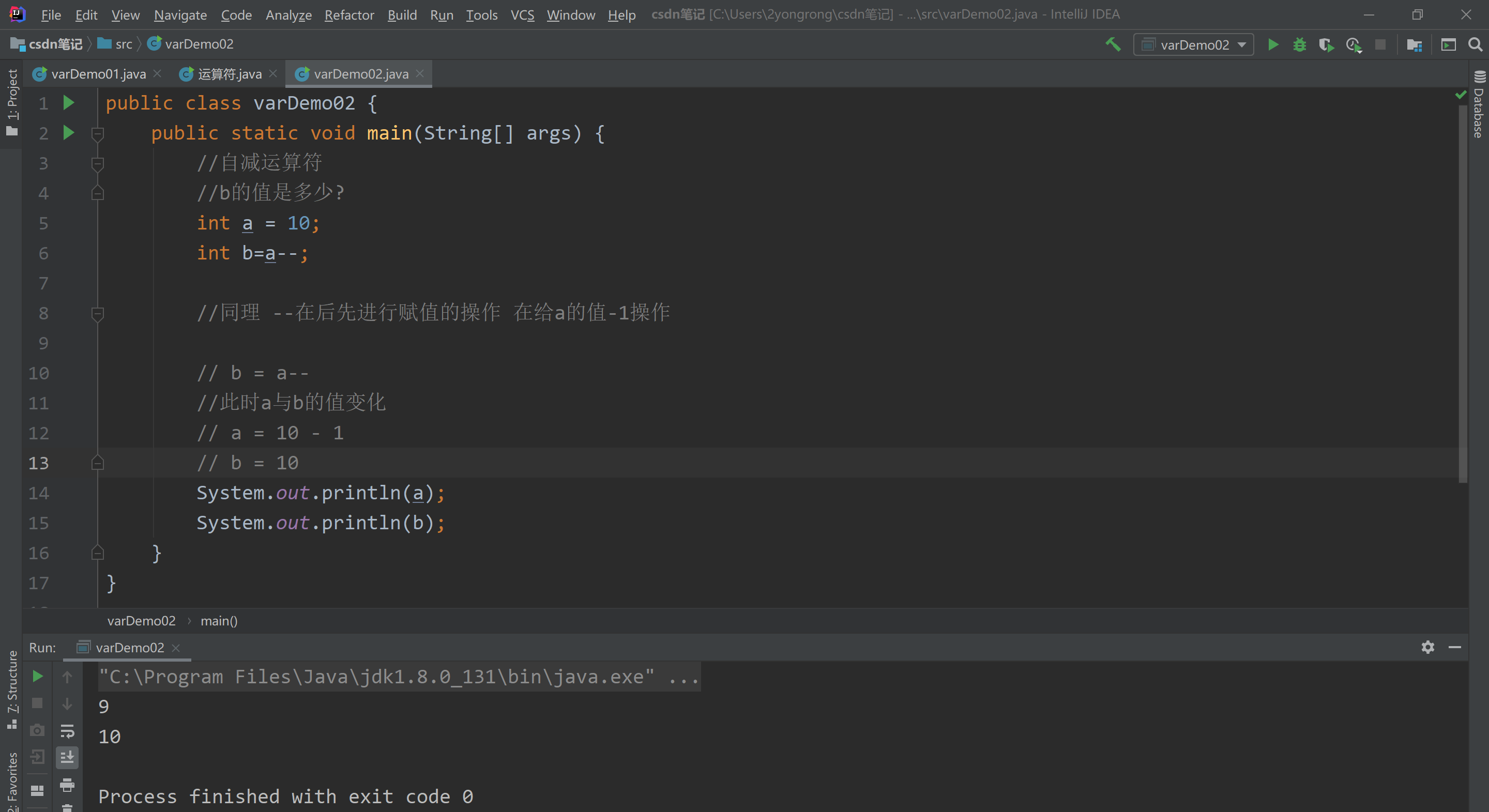我们把运算符写到前面 结果变化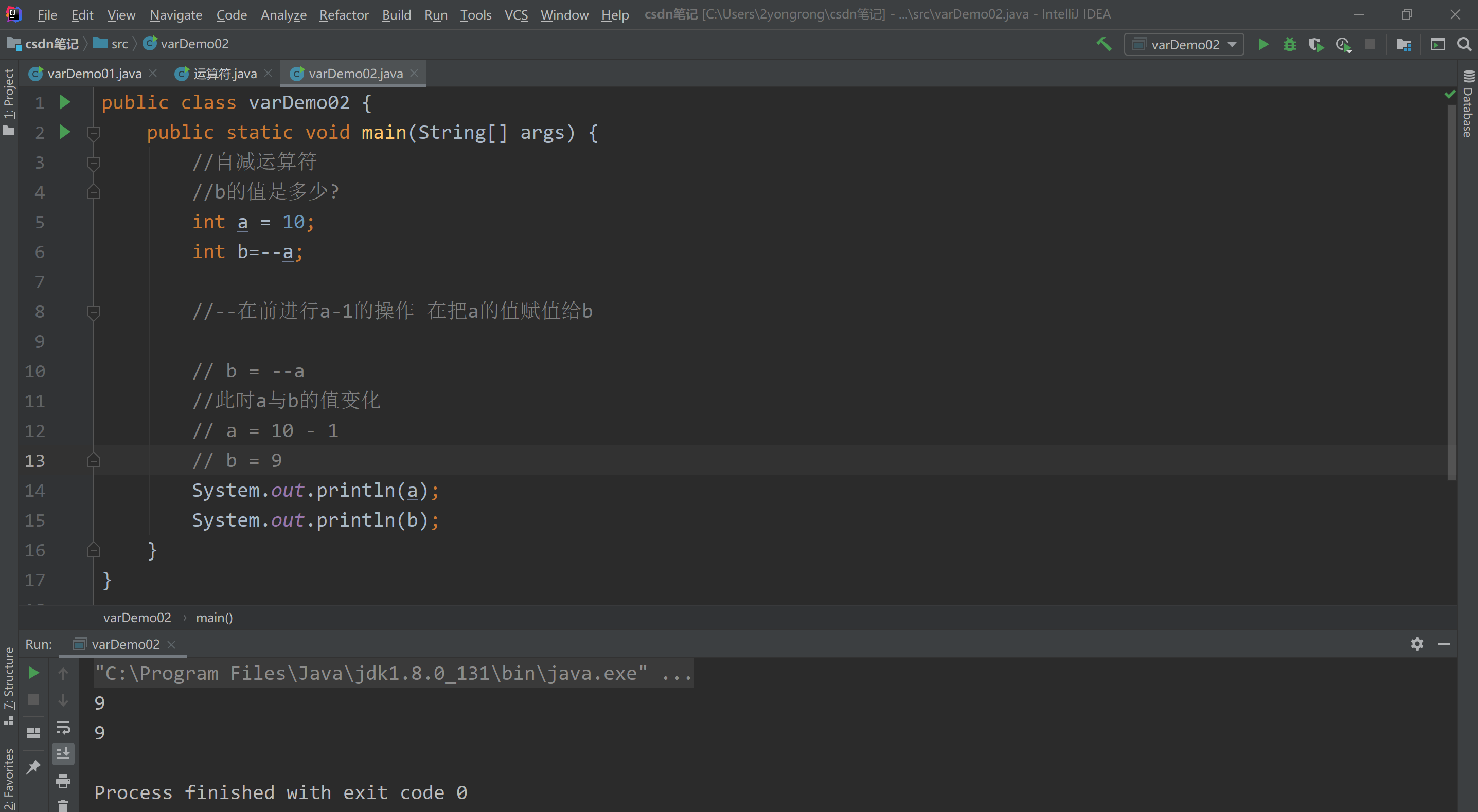## 1.关系运算符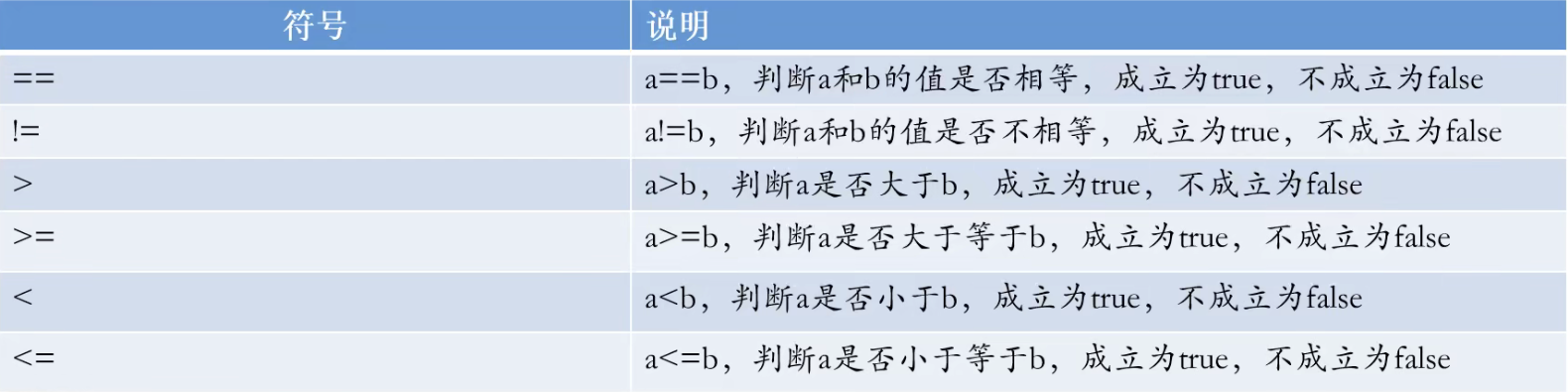注意事项:关系运算符的结果都是boolean类型，要么是true 要么是false。千万不要把’'误写成’=‘，’‘是判断是否相等的关系,‘=’是赋值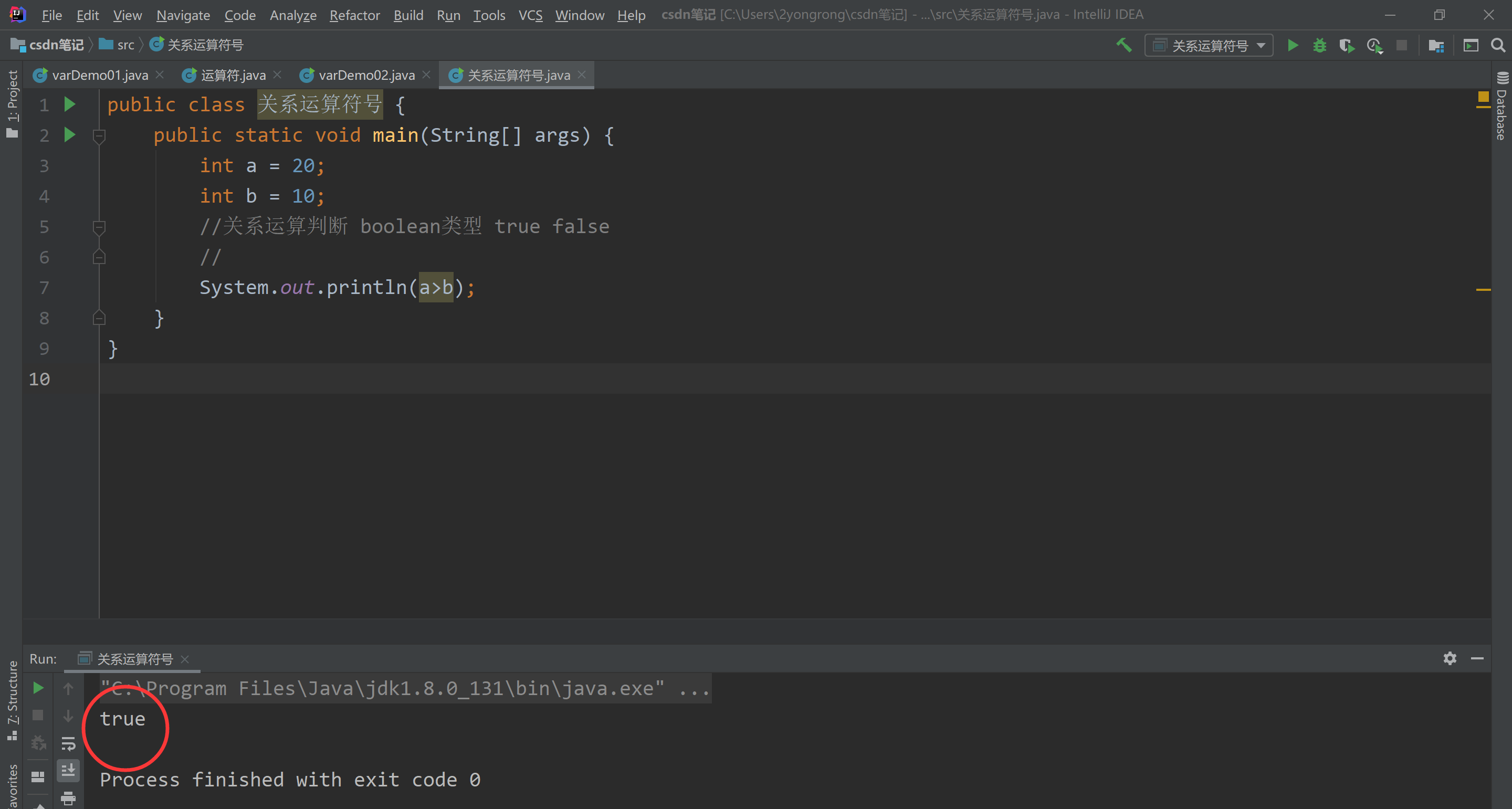## 判断值是否相等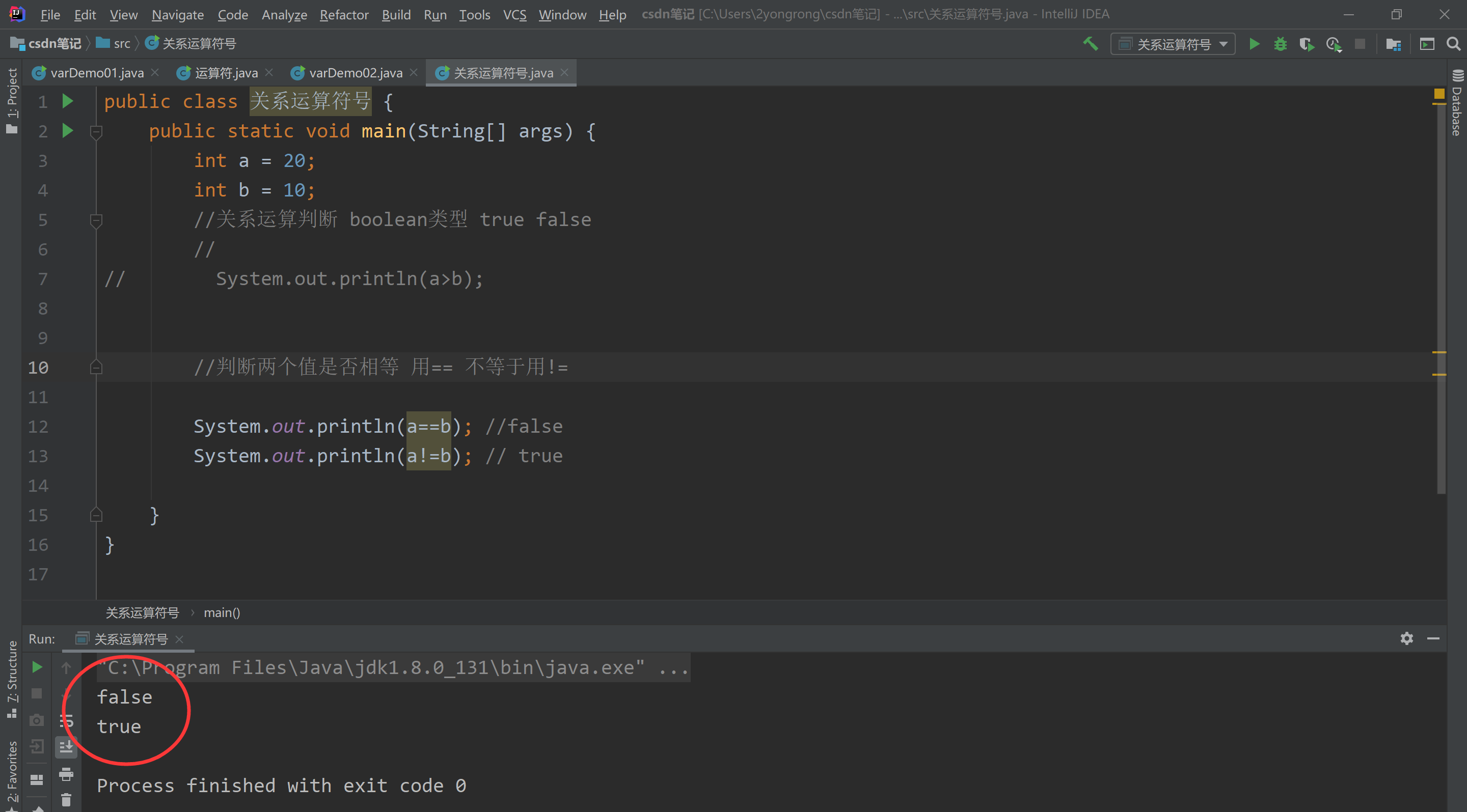## 1.2逻辑运算符概述

逻辑运算符把各个运算的关系表达式连接起来组成一个复杂的逻辑表达式，以判断程序中的表达式是否成立，判断的结果是true或false。
在数学中，一个数据x，大于3，小于6，我们可以这样来进行表示:3<x<6。在Java中，需要把上面的式子先进行拆解，再进行合并表达。
拆解为: x>3和x<6
合并为: x>3&&x<6
&&其实就是一个逻辑运算符。
我们可以这样说，逻辑运算符，是用来连接关系表达式的运算符。当然，逻辑运算符也可以直接连接布尔类型的常量或者变量。

## 1.2.1逻辑运算符

逻辑运算符把各个运算的关系表达式连接起来组成一个复杂的逻辑表达式，以判断程序中的表达式是否成立，判断的结果是true或false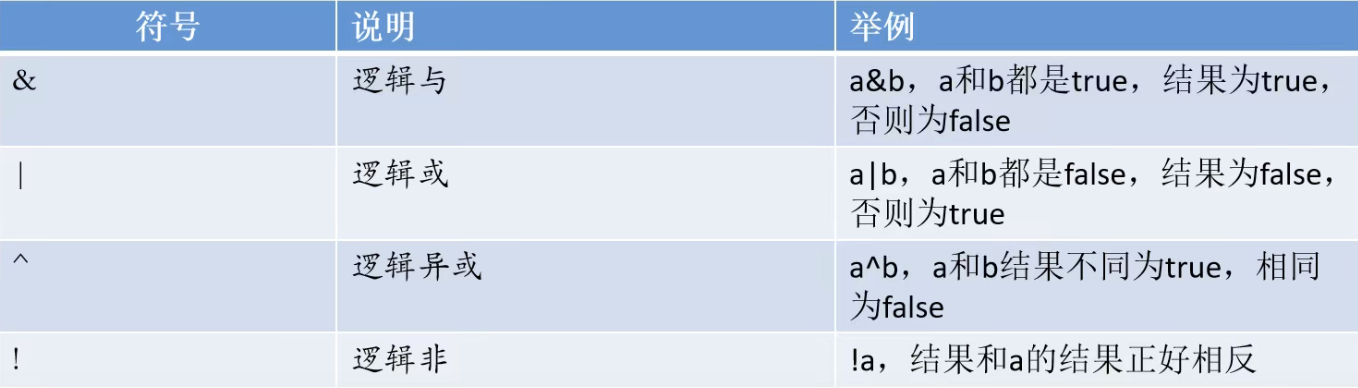&逻辑与表达式

 //&
System.out.println("&并且");
System.out.println(true&true);
System.out.println(true&false);
System.out.println(false&false);
int a = 10;
int b = 20;
int c = 30;
//a>b false b>c false
System.out.println(a>b&b>c); //false
//a<b true b<c true
System.out.println(a<b&b<c);//true
//a<b true b>c false
System.out.println(a<b&b>c);//false



逻辑异或表达式 与逻辑非表达式

System.out.println("-----------逻辑异或----------");
System.out.println(true^false);//true
System.out.println(true^true);//false
System.out.println(!true);//相反值 //false


## 1.2.2短路逻辑运算符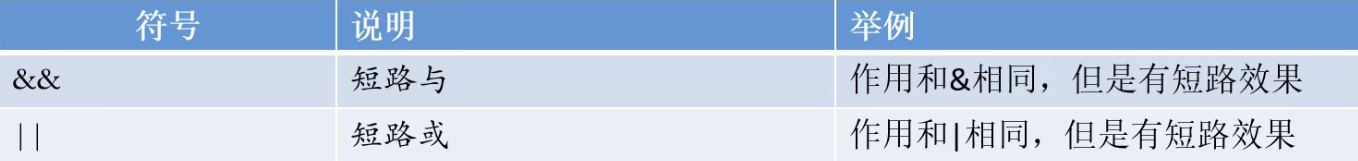只要有一个表达式的值为false，那么结果就可以判定为false了，没有必要将所有表达式的值都计算出来，短路与操作就有这样的效果，可以提高效率。同理在逻辑或运算中，一旦发现值为true，右边的表达式将不再参与运算。逻辑与&无论左边真假，右边都要执行
短路与&&，如果左边为真，右边执行;如果左边为假，右边不执行。逻辑或|，无论左边真假，右边都要执行。短路或，如果左边为假，右边执行;如果左边为真，右边不执行。3>6&&4<10
3>6 false

int a=10;int b=20;
System. out. println((a++>100)&(b++>100)) ;
System. out. println("a:"+a) ;
System. out. println("b:"+b);


逻辑与 与短路与的区别 &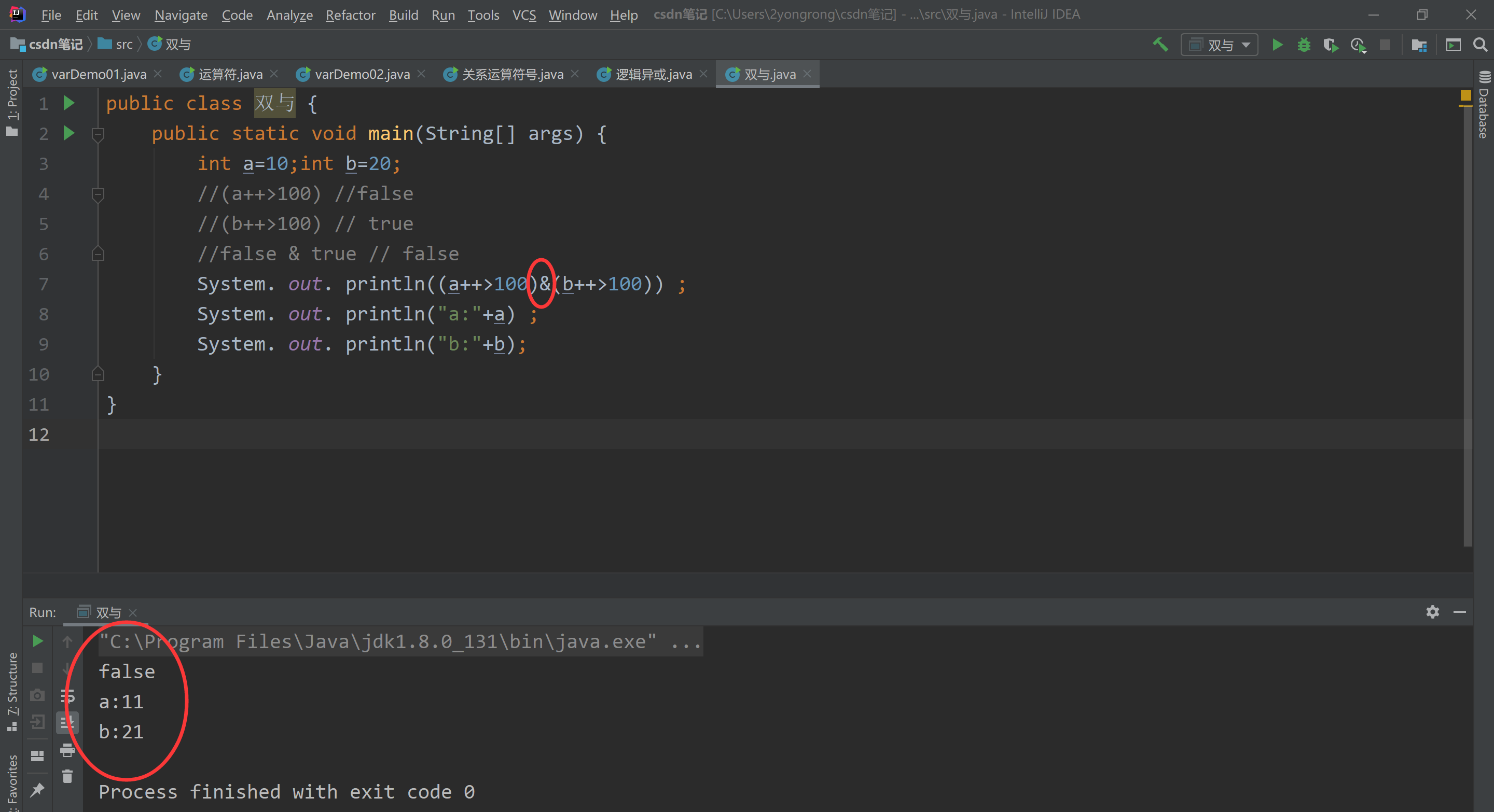int a=10;
int b=20;
// 左边 & 右边 都必须为true 则返回结果是为true
//a++ > 100 返回为false  (b++>10)true
// 左边返回false 右边不会执行
System. out. println((a++>100)&&(b++>100)) ;
System. out. println("a:"+a) ;
System. out. println("b:"+b);


逻辑与 与短路与的区别 &&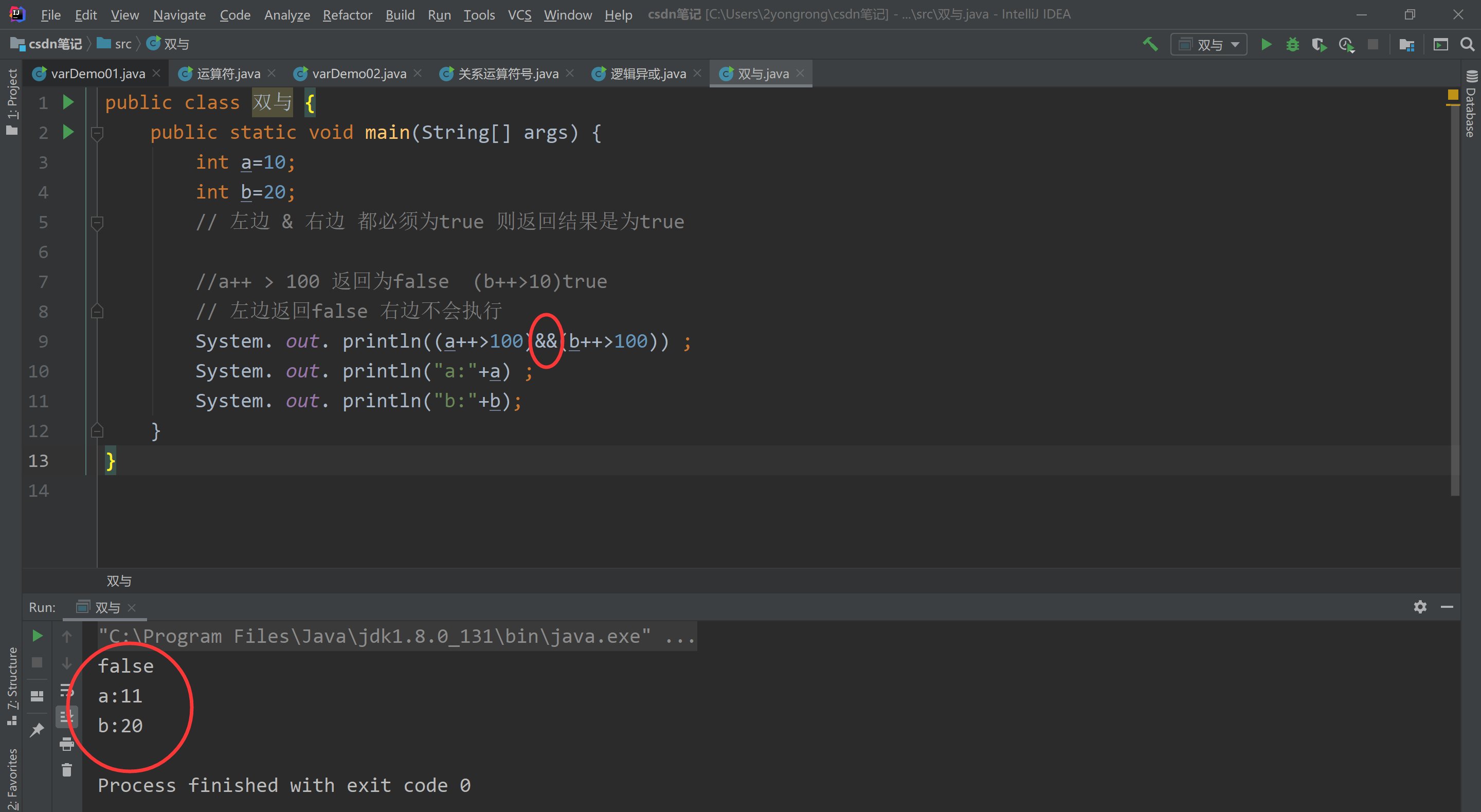逻辑或 与短路或的区别 |

int a=10;
int b=20;
System.out.println("短路或");
//或者--左边喝右边只要 有一个返回true 最终结果就返回true
//(a++>1) true  b++>100 false
System. out. println((a++>1)|(b++>100)) ;
System. out. println("a:"+a) ;
System. out. println("b:"+b);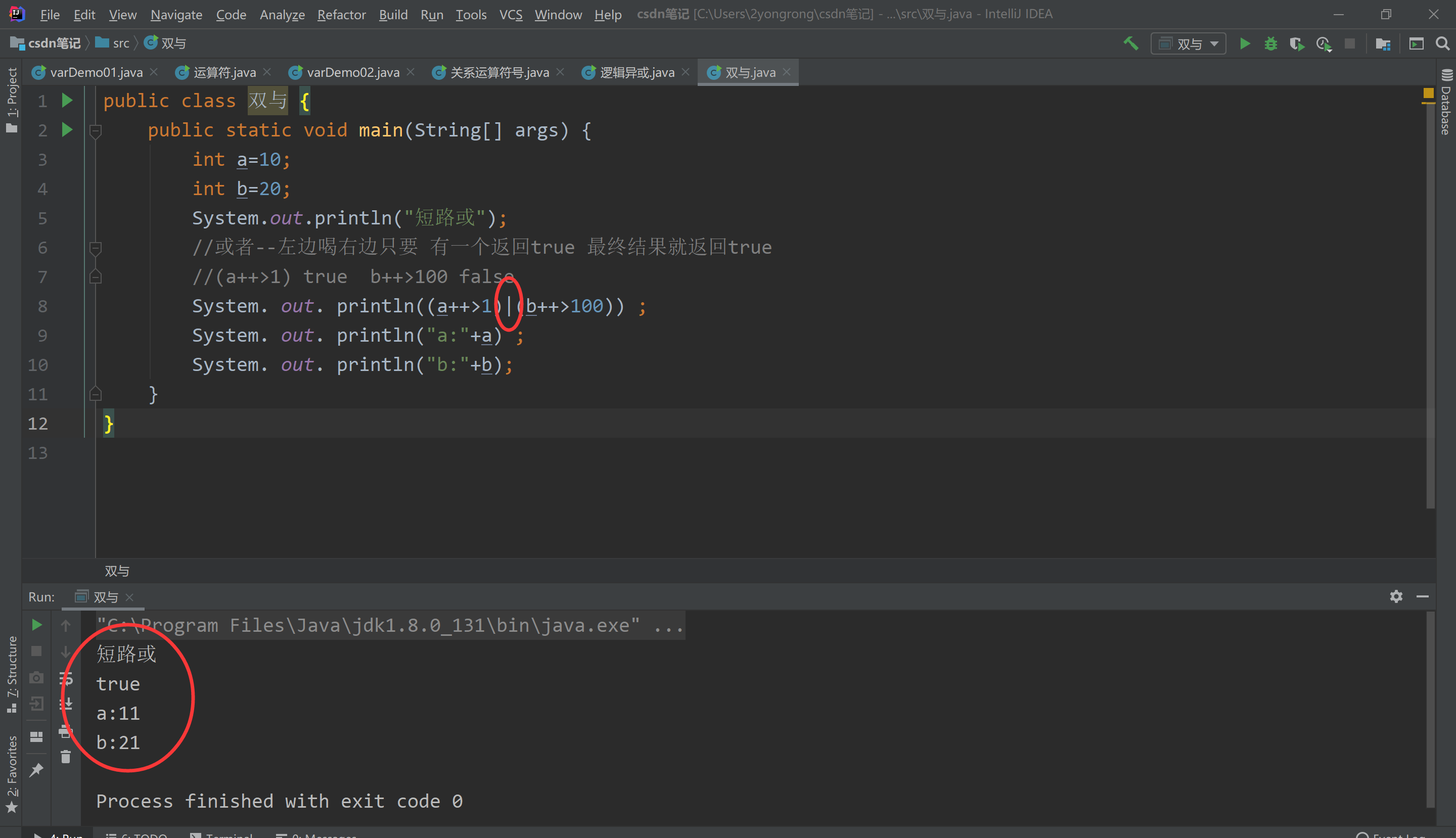逻辑或 与短路或的区别 ||

int a=10;
int b=20;
System.out.println("短路或");
//逻辑或 左边为true 不会判断右边 就不会执行 就不会加减
//(a++>1) true  b++>100 false
System. out. println((a++>1)||(b++>100)) ;
System. out. println("a:"+a) ;
System. out. println("b:"+b);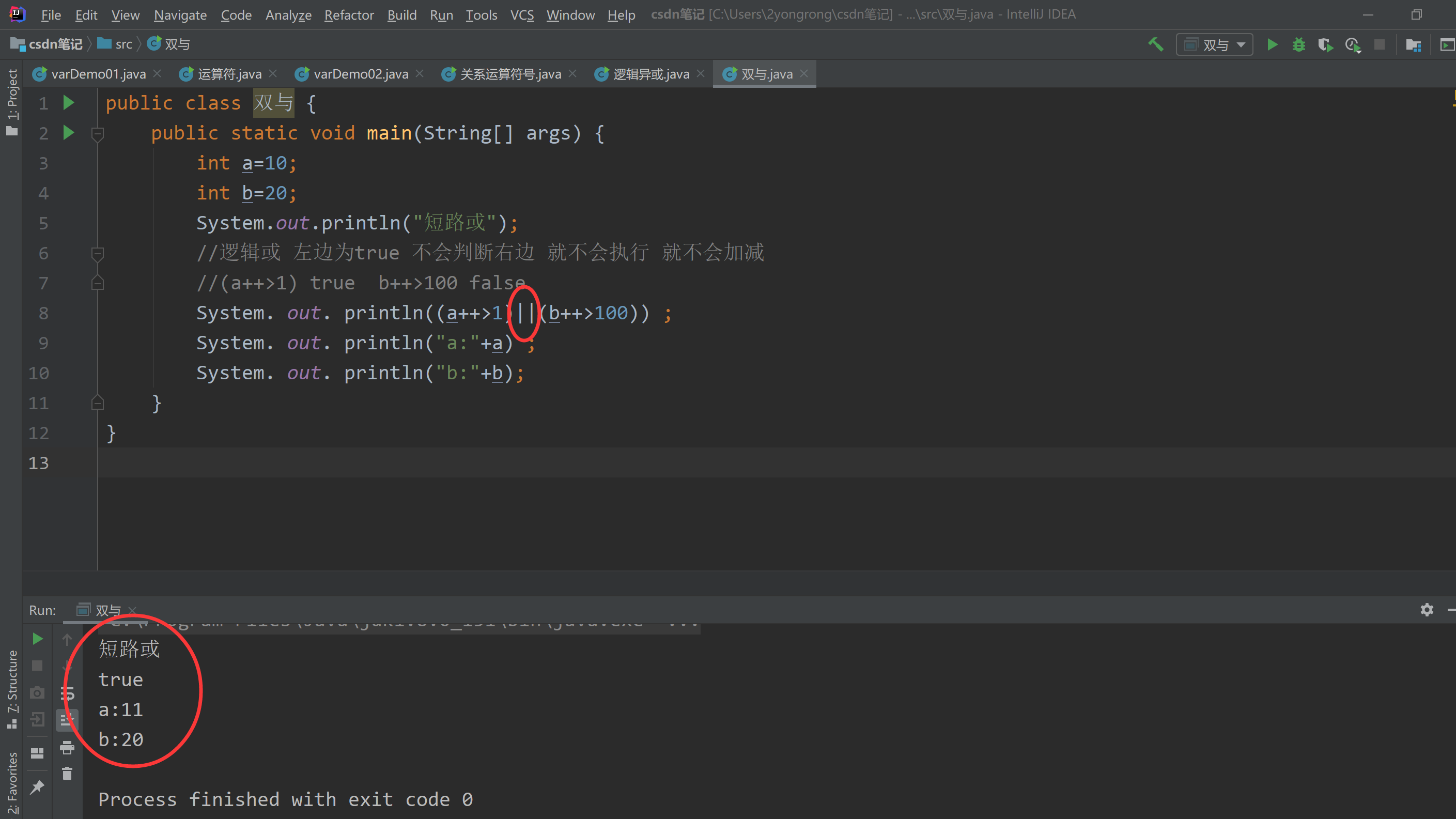展开全文• Java语言支持如下运算符： 优先级>>()括号优先 在进行复杂运算的时候我们可以多打一些括号 算术运算符和赋值运算符 实例1： package JavaBasics; public class Operater { public static void main...

# 基本运算符

## 运算符

• Java语言支持如下运算符： 优先级>>()括号优先 在进行复杂运算的时候我们可以多打一些括号• 算术运算符和赋值运算符

实例1：

package JavaBasics;

public class Operater {
public static void main(String[] args) {
// 二元运算符
//ctrl+：复制当前行到下一行
int a = 10;
int b = 20;
int c = 25;
int d = 25;

System.out.println(a+b);
System.out.println(a-b);
System.out.println(a*b);
System.out.println(a/b);
//a和b都是整形，a/b的结果也是整形；结果0.5将会把小数点之后的数舍去，注意这里不四舍五入
System.out.println(a/(double)b); //强转其中一个数为双精度型
}
}


实例2：

package JavaBasics;

public class Operater02 {
public static void main(String[] args) {
long a = 1221551155454L;
int b = 123;
short c = 8;
byte d = 8;

//优先级  byte>>short>>char>>int>>long>>float>>double

//优先级在long之前的运算数，运算数中有long则结果为long，没有long则为int
System.out.println(a+b+c+d); //long
System.out.println(b+c+d);  //int
System.out.println(c+d);   //int
//优先级在long之后的运算数参与运算结果为long之后的运算数类型
System.out.println((double) c+d);   //double
}
}

• 关系运算符

实例：

package JavaBasics;

public class Operater03 {
public static void main(String[] args) {
//关系运算符返回的结果：正确，错误 布尔值
//if

int a = 10;
int b = 20;
int c = 22;

//取余，模运算
System.out.println(c%b); //21 / 10 = 2 ... 1

System.out.println(a>b);   //boolean
System.out.println(a<b);   //boolean
System.out.println(a==b);  //boolean
System.out.println(a!=b);  //boolean
}
}

• 逻辑运算符

实例：

package JavaBasics;
//逻辑运算符
public class Operator05 {
public static void main(String[] args) {
//与（and） 或（or） 非（取反）
boolean a = true;
boolean b = false;

System.out.println("a && b:"+(a&&b)); //逻辑与运算：两个变量都为真，结果才为真
System.out.println("a || b:"+(a||b)); //逻辑或运算：两个变量结果都为假结果，才为假
System.out.println("! (a && b):"+(!(a&&b))); //如果是真则为假，如果是假，则为真

//短路运算
in = 5;
boolean d = (c<4)&&(c++<4); //短路 只执行前半部分，后半部分没有执行的意义
System.out.println("==============");
System.out.println(d);
System.out.println(c);
}
}

• 位运算符

实例：

package JavaBasics;

public class Operater06 {
public static void main(String[] args) {
/*
A = 0011 1100
B = 0000 1101
-----------------
A&B = 0000 1100
A|B = 0011 1101
A^B = 0011 0001
~B=1111 0010

2*8 = 16  2*2*2*2
效率极高！！！
<< *2 左移
>> /2  右移

0000 0000   0
0000 0001   1
0000 0010   2
0000 0011   3
0000 0100   4
0000 1000   8
0001 0000   16

*/
}
}

• 条件运算符

实例：

package JavaBasics;

//三元运算符
public class Operater08 {
public static void main(String[] args) {
//x ? y : z
//如果x==true，则结果为y，否则为z

int score=60;
String type=score<60?"不及格":"及格"; //必须掌握
// if
System.out.println(type);

}
}

• 扩展赋值运算符

实例：

package JavaBasics;

public class Operater04 {
public static void main(String[] args) {
//++  --  自增，自减 一元运算符
int a = 3;
int b = a++; //a++ 先赋值在++
System.out.println(a);
int c = ++a; //++a 先++再赋值

System.out.println(a);
System.out.println(b);
System.out.println(c);

//幂运算 2^3 2*2*2 = 8
//Math 数学类 很多运算我们会使用一些工具类来操作！
double pow = Math.pow(2, 3); //Math. pow然后alt+enter组合键引入局部变量
System.out.println(pow);
}
}

• 字符串连接符

实例：

package JavaBasics;

public class Operater07 {
public static void main(String[] args) {
int a = 10;
int b = 20;

a+=b; //a=a+b

System.out.println(a);

//字符串连接符 + , String
System.out.println("字符串连接符应用："+(a+b));
//输出的（a+b）为字符
System.out.println(a+b);
System.out.println(""+a+b);
System.out.println(a+b+"");
}
}

展开全文• 一、Java运算符分类 1.算术运算符：+ - * / % ++ ++、--运算符的四种情况： （1）i++：使用 i之后，i的值再加1，即先取值，后自加。等价于i=i+1. （2）++i：先使I加1，然后再使用i，即先自加，后取值。等价于 i =...

### 一、Java运算符分类

##### 1.算术运算符：+ - * / % ++
          ++、--运算符的四种情况：
（1）i++：使用 i之后，i的值再加1，即先取值，后自加。等价于i=i+1.
（2）++i：先使I加1，然后再使用i，即先自加，后取值。等价于 i = i+1.
（3）i--：使用i之后，i的值再减1，即先取值，后自减。等价于i=i-1；
（4）--i：先使I减1，然后再使用i，即先自减，后取值。等价于i=i-1.
注意：++和--运算符只能用于变量，不能用于常量和表达式。因为++和--蕴含着赋值操作。++和--运算符经常用于循环程序设计中。

##### 2.赋值运算符：= （符合符号运算符+= -+ *= /=）
       作用：设置变量的值，即将一个数据赋给一个变量，实际上是将特定的值写到变量所对应的内存单元里。

##### 4.按位运算符：|（位或） &（位与） ^（位异或） ~（按位求反） >>（保留符号位右移） >>>（不保留符号位右移）

<<<
作用：对于整数数值的二进制表示进行计算。

### 二、运算符的优先级：

    1.[] ()
2.++ -- ! ~
3.* / %
4. + -
5.>> >>>  <<
6.> >= < <=
7.== !=
8.&
9.^
10. |
11.&&
12.||
13. ?
14. (最低) = *= /= += -= <<= >>= >>>= &= ^=


### 三、Java中的语句

 1.顺序结构
2.分支结构
if else 、switch case
3.循环结构
while 、do while、for


### 四、跳转语句

 （return、break、continue）
return 语句用来从当前方法中退出，返回到调用该方法的语句处，并从紧跟该语句的下一条语句继续执行。如果在main（）方法中执行return语句，则使程序0返回到java运行系统，因为java运行系统调用了main（）方法。
要想从程序的任意地方直接一步到位的退出程序，而不是返回到调用该方法的语句处，可以使用System.exit(0)方法。

展开全文• 运算符：算术运算符 运算符 运算 范例 结果 + 正号 +3 3 - 负号 b=4;-b -4 + 加 5+5 10 - 减 6-4 2 * 乘 3*4 12 / 除 5/5 1 % 取模(取余) 7%5 2 ++ 自增(前):先运算后取值 a=2;b=++a a=3;b=3 ...开发语言
• 1.运算符和表达式：1、运算符：对常量或者变量进行操作的符号2、表达式：用运算符把常量或者变量连接起来符合Java语法的式子就可以称为表达式。不同运算符连接的表达式是不同的表达式。//举例说明：int a = 10;int b...
• 运算符是对常量或者变量进行操作的符号，Java运算符有六类：算数运算符、赋值运算符、自增自减运算符、关系运算符、逻辑运算符，三元运算符、用运算符把常量或者变量连接起来符合java语法的式子就可以称为表达式。...
• Java运算符之比较运算符 比较运算符用于比较两个变量或常量的大小，比较运算的结果是一个布尔值(true或false)。Java支持的比较运算符如下： 1.’ >:’ 大于，只支持左右两边的操作数是数值类型。如果前面变量的值...
• 特点：使用比较符合连接的表达式无论是简单表达式还是复杂表达式，结果都是boolean类型 注意： ==比较的两个数据值是否相等，不要写成=（赋值运算符） */ class OperatorDemo{ public static void main(String...开发语言
• Java语言提供许多操作符。操作符是特殊的符号(symbol)，它对一个或者两个、三个的操作数进行运算，然后返回一个结果，最简单的就像我们一年级学到的+ -号。一般地，可以将运算符分为四大类：算数运算符、位运算符、...
• 一元运算符：只需要一个数据就可以进行操作的运算符。例如：取反！、自增++、自减-- 二元运算符：需要两个数据才可以进行操作的运算符。例如：加法+、赋值= 三元运算符：需要三个数据才可以进行操作的运算符。 格式...
• 短路逻辑运算符：一但符合条件，后面的判断不走 二、逻辑运算符 逻辑运算符：&、|、^、! 案例代码 public class test { public static void main(String[] args) { // 定义变量 int i = 10; int j ...
•flutter
• 运算符包括：“&”、“|”、“~”、“^”和移位运算符“>>”、“”、“>>>”，7个位运算符。按位与的运算符用符号“&”表示，按位与参与运算的数字，低位对齐，高位不足的补0，当对应的二进制位都为1时，计算结果...
• 赋值运算符“=”，是 JAVA 中使用最多的运算符，作用是将一个值(字面量值、变量值、 表达式计算结果)复制一份给另一个变量。赋值运算符常见形式： 变量 = 表达式; a = 1+1; //先计算表达式 1+1，然后将结果值赋给...
• Java中的复合运算符，比如 += 、-= 等，一般来说，a = a + b 与 a += b 是等价的，但实际上从严格意义上来说，这二者之间并不等价，还是有一定区别的。比如:public class TestCompositeOperator {public static void...
• java中含有的运算符（算数、比较、逻辑、条件、位运算） java中的算数运算符 java中的算数运算符都有+ - * / % ++ – + 加法，也可是字符串的连接 - （减法） *（乘法） /（除法） public class Demo { public ...
• ## java三目运算符

万次阅读 2018-01-12 15:49:18
写在前面： 三目运算符是我们经常在代码中使用的，a= (b=...前段时间遇到（一个由于使用三目运算符导致的问题，其实是因为有三目运算符和自动拆箱同时使用（虽然自动拆箱不是我主动用的）。 一、三目运算符 对于条
• &&（并且）： 全都是true,才是true;否则就是false ||（或者）： 至少一个是true,就是true;...1.逻辑运算符只能用于boolean值。 2.与、或需要左右各自有一个boolean值，但是取反只要有唯一的一个boolean值即可.
• JAVA 三元运算符了解运算符 一元运算符：只需要一个数据就可以进行操作的运算符。例如：取反!、自增++、自减– 二元运算符：需要两个数据才可以进行操作的运算符。例如：加法+、赋值= 三元运算符：需要三个数据才...
• Java 运算符 算数运算符、赋值运算符、关系运算符、逻辑运算符、位运算符、三元运算符
• 赋值运算符 赋值运算符分为两种 ①基本赋值运算符：就是一个等号“=”，代表把右侧的数据交给左侧的变量。 比如： int a = 30; ②复合赋值运算符： +=a += 1 相当于 a = a + 1 -= b-= 4 相当于 b = b - 4 *= ...算法
• 三元运算符概念运算符：进行特定操作的符号如：+ – * /表达式：用运算符连起来的式子叫表达式在Java中分为：算数运算符、赋值运算符、比较运算符、逻辑运算符、三元运算符算数运算符基础用法public static voidmain...
• 赋值运算符以下是Java语言支持的赋值运算符-运算符描述例=简单的赋值运算符。将值从右侧操作数分配给左侧操作数。C = A + B将A + B的值赋给C+ =添加AND赋值运算符。它将右操作数添加到左操作数，并将结果分配给左...
• 算术运算符都是双目运算符，即连接两个操作数的运算符。优先级上，*、/、％ 具有相同运算级别，并高于 +、-（+、- 具有相同级别）加减乘除，很简单就不用说了，大家都懂,就这个取余要求必须是两个整型数字才行++ 或 ...
• 一、JAVA运算符。1.赋值运算符赋值就是把一个变量的值赋给另一个变量。语法： 变量名=表达式例如 n = m + 52.算术运算符算术运算符是数学中常用的加、减、乘、除、余。(+、-、*、/、%)复合算术运算符：+=、-=、*=...
• 简化运算符代码：x＝x＋y 可以变成x＋＝y求模(％)＋＋(自增)〈＋＋变量：表示先进行变量的自增1，而后再利用变量进行操作〉〈变量＋＋：表示先使用变量的内容进行计算，而后自增〉，－－(自减)int a = 3;int b = a++......